Next: Figures Up: H3: Laying Out Large Previous: References

# Appendix A: Layout Derivation

The H3 layout method operates in two passes: in the bottom-up pass we find an approximate radius for each hemisphere and in the top-down pass we place children on the surface of their parent hemisphere. In this Appendix we present a detailed derivation of the radius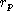of a parental hemisphere and the triple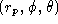needed to place a child hemisphere on the surface of that parental hemisphere. For each step of the derivation, we show first the euclidean then the hyperbolic result.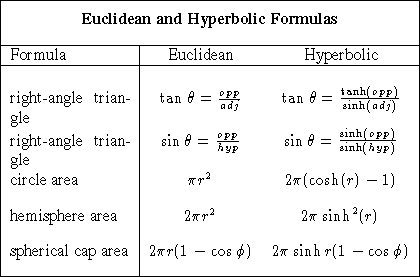Bottom-up pass: We compute a target surface area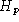of a hemisphere at level p by summing the areas of the disks at the bottom of the child hemispheres at level p+1. This target surface area is only an approximation for three reasons. First, the surface area of a spherical cap is greater than the disk on which it lies. (We use the disk approximation since its area does not depend on the as yet unknown parental radius, which we would need to compute the area of the spherical cap.) Second, even an optimal circle packing of the disks of the sphere leaves uncovered gaps between the circles. Third, our circle packing is known to be suboptimal: circles may use less vertical space than their alloted band allows, and there may be unused horizontal space in the outermost band. These issues are discussed in more detail in Section 3.

All three reasons lead to an estimate which is less than the necessary area. We use an empirically derived area scaling factor to ensure that collisions do not occur. The other parameter in our implementation is the surface area alloted for hemispheres of leaf nodes. The layout would be too dense if the leaf nodes touched, so we generally specify a larger area than a hemisphere that would exactly fit around the geometric representation of a node. These two parameters control the density of the layout.

The euclidean derivation of the target hemisphere surface area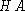at level p is straightforward.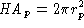, so euclidean radiuswould be. The relationship between the parent and child hemispheres is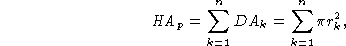where DA is the area of the disk at the bottom of a child hemisphere and n is the number of children at level p+1.

When we use the hyperbolic area formula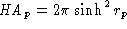, the hyperbolic radius of the parental hemisphere is.

The parent-child relationship becomes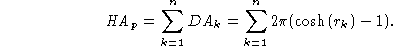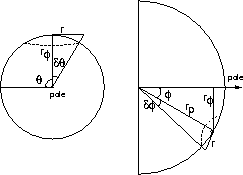Figure 7: We find spherical coordinates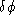and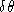for placing a child hemisphere on the surface of a parent hemisphere with radius.Top-down pass: We use the radiusof the parent hemisphere to compute the remaining two spherical coordinatesand, which specify the position of the child hemisphere at level p+1. We computeandcumulatively, starting from the pole at the top of the hemisphere. The children are laid out in concentric bands surrounding the pole at the top of the hemisphere. The total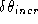angle between child n and child n+1 on band j is the sum of the angles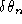and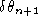, which depend on the radii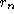and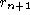of the children. We need to derive the anglegiven some r as in Figure 7. An angledepends on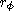, the radius of the spherical cap at. When we use the euclidean radius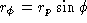and the euclidean right-angle triangle formula we find the euclidean angle. If we instead use the hyperbolic radius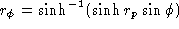and the hyperbolic right-angle triangle formula we find the hyperbolic angle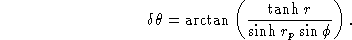We substituteandto obtain our total: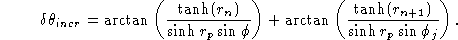If the cumulative angle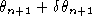is greater than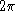, we drop down to the next band j+1 and resetto 0. (The very first child is a singular case, since the band is just a spherical cap.) Theangle between a child on one band and the next depends on the radius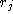of the largest child in band j and the radius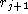of the largest child in band j+1. In our current circle packing approach, we know that the first child in each band will have the largest radius, since we lay out the children in descending sorted order.

We thus need to derive the anglegiven some r, as in the bottom of Figure 7. The euclidean anglecorresponding to r is simply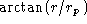. We substitute the hyperbolic formula for right-angle triangles to find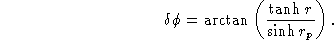We substituteandto obtain our total: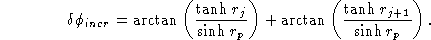Armed with the triple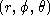, we can center the child hemisphere in the appropriate spot on the parent hemisphere.Next: Figures Up: H3: Laying Out Large Previous: References

Tamara Munzner Tue Jul 22 08:42:41 PDT 1997